Question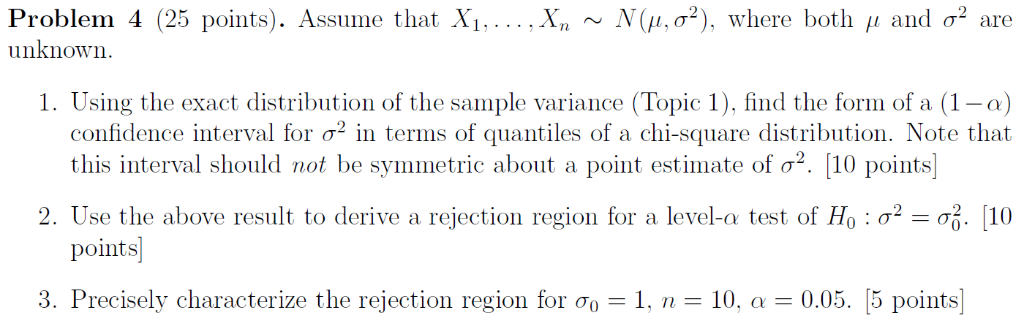DISTRIBUTION OF SAMPLE VARIANCE: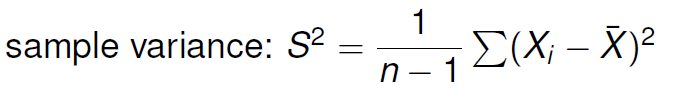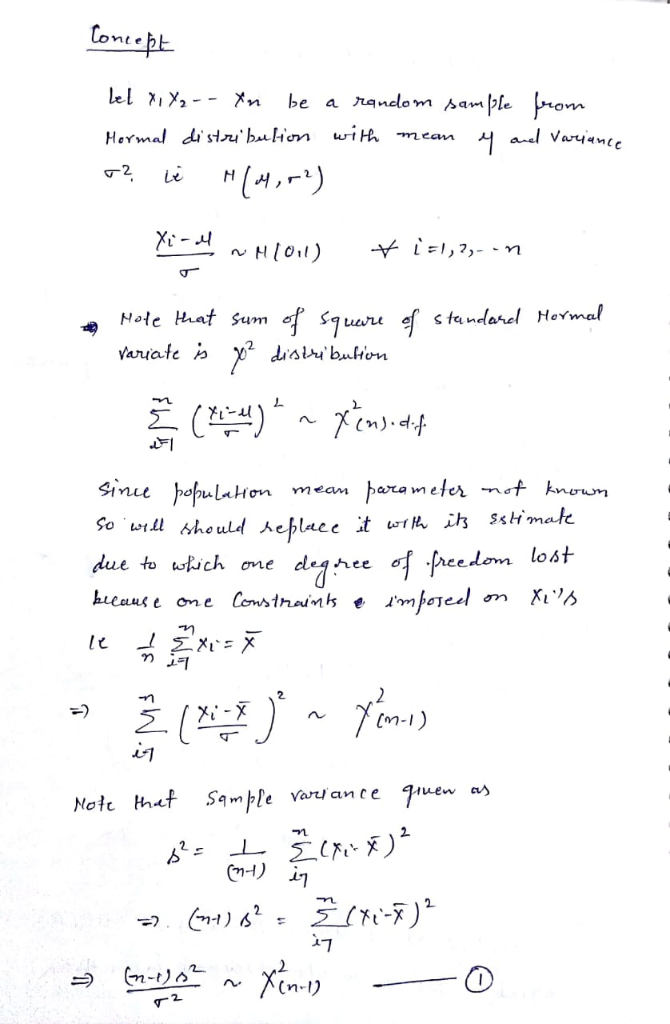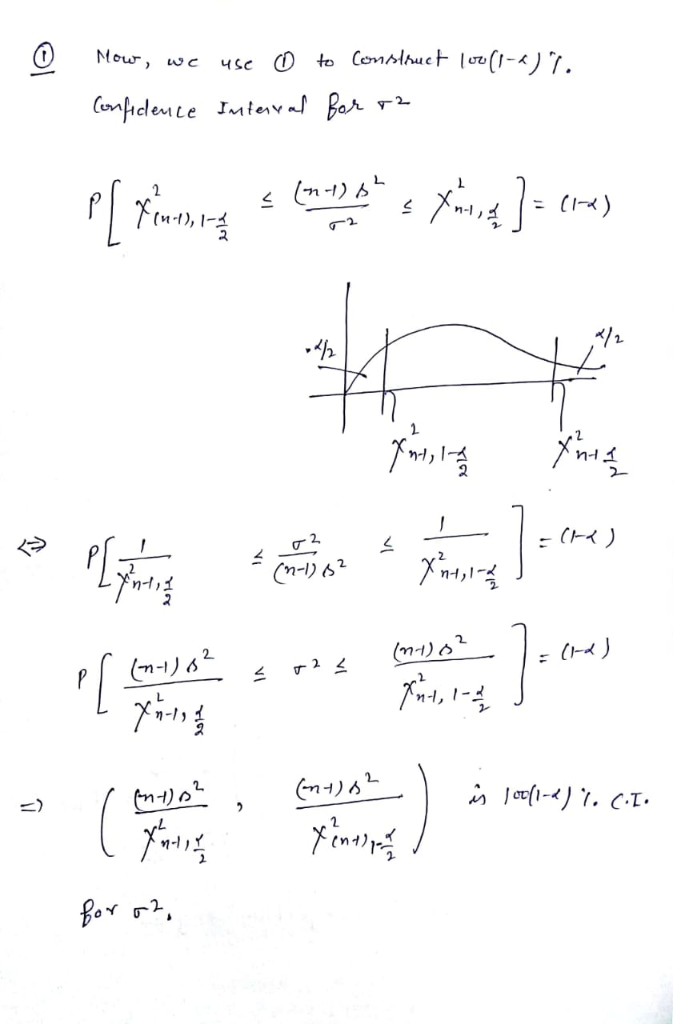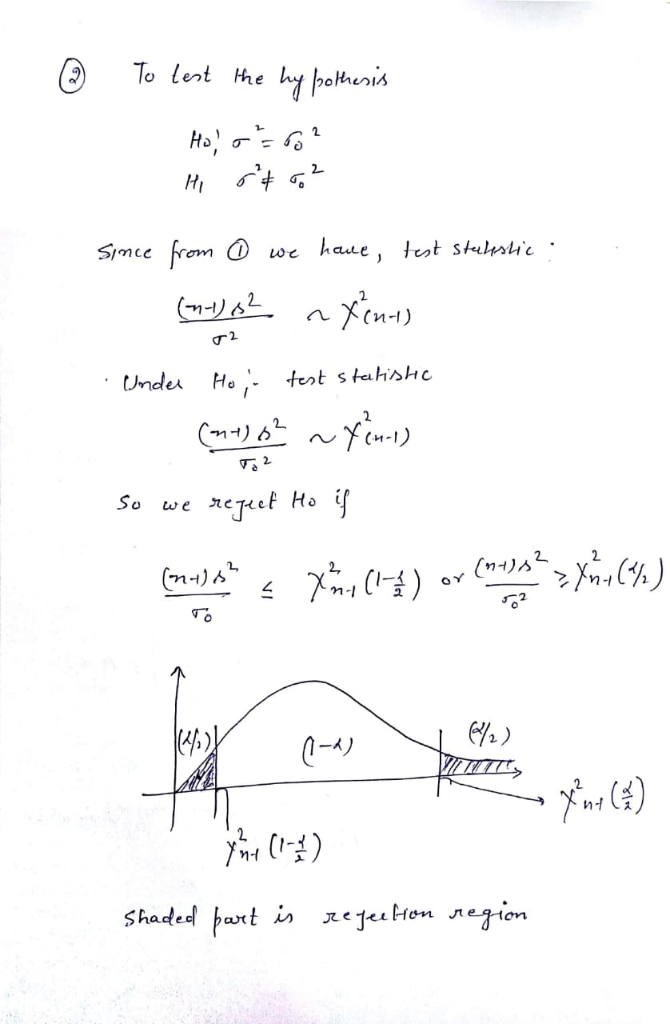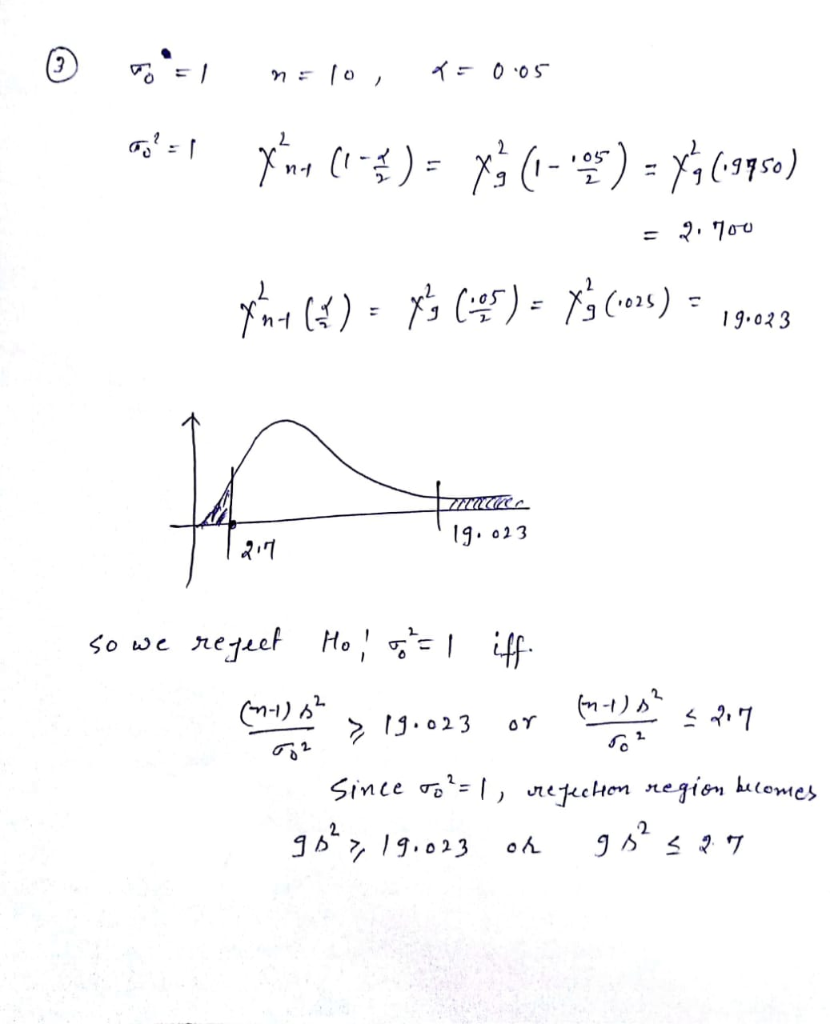#### Earn Coins

Coins can be redeemed for fabulous gifts.

Similar Homework Help Questions
• ### Let Xi, ,Xn be a sample from N(μ, σ2) and assume that both parameters are unknown. Consider testi...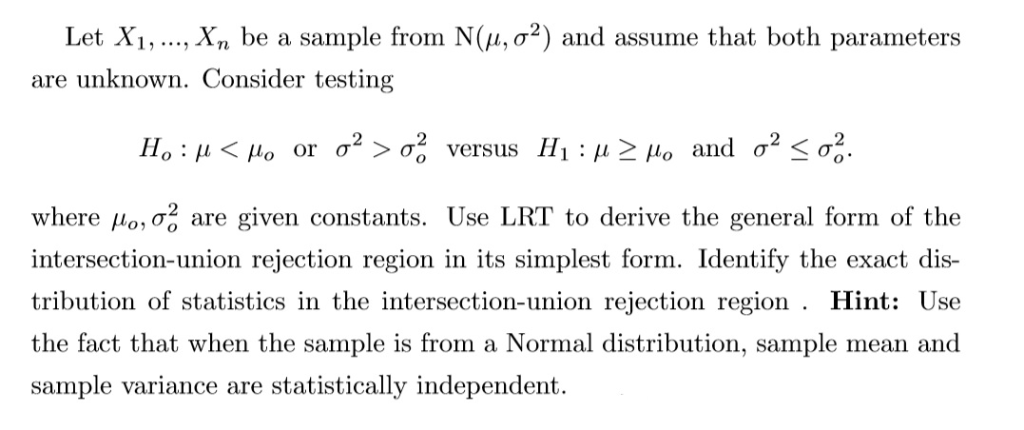Let Xi, ,Xn be a sample from N(μ, σ2) and assume that both parameters are unknown. Consider testing where μοισ., are given constants. Use LRT to derive the general form of the intersection-union rejection region in its simplest form. Identify the exact dis- tribution of statistics in the intersection-union rejection region . Hint: Use the fact that when the sample is from a Normal distribution, sample mean and sample variance are statistically independent. Let Xi, ,Xn be a sample from...

• ### Let X1,X2, , Xn be a random sample from a normal distribution with a known mean μ (xi-A)2 and variance σ unknown. Let ơ-- Show that a (1-α) 100% confidence interval for σ2 is (nơ2/X2/2,n, nơ2A-a/2,n)...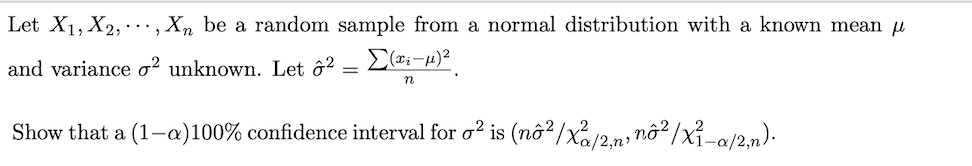Let X1,X2, , Xn be a random sample from a normal distribution with a known mean μ (xi-A)2 and variance σ unknown. Let ơ-- Show that a (1-α) 100% confidence interval for σ2 is (nơ2/X2/2,n, nơ2A-a/2,n). Let X1,X2, , Xn be a random sample from a normal distribution with a known mean μ (xi-A)2 and variance σ unknown. Let ơ-- Show that a (1-α) 100% confidence interval for σ2 is (nơ2/X2/2,n, nơ2A-a/2,n).

• ### . Let Yi, ,Ý, be a sample from N(μ, σ2) distribution, where both μ and σ2...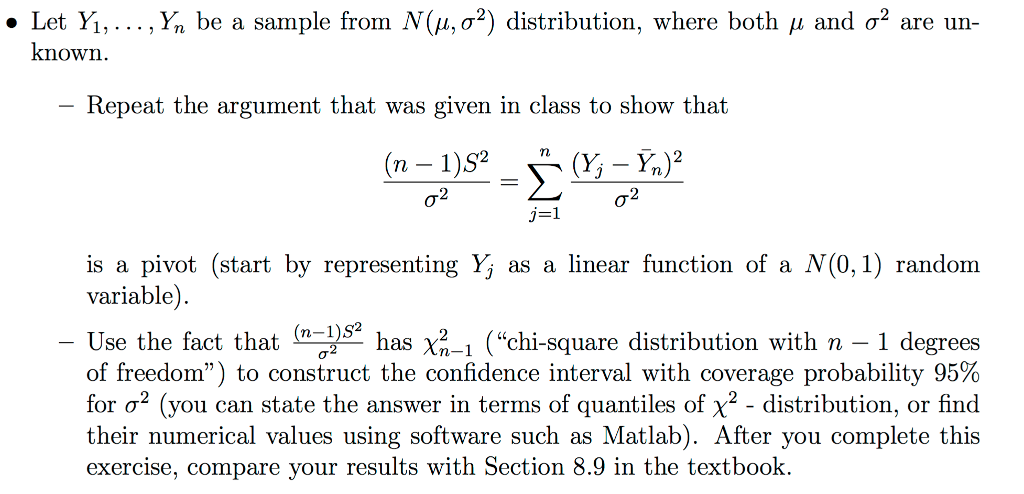. Let Yi, ,Ý, be a sample from N(μ, σ2) distribution, where both μ and σ2 are un known Repeat the argument that was given in class to show that is a pivot (start by representing Yj as a linear function of a N(0, 1) random variable). Use the fact that (n-pe, of freedom") to construct the confidence interval with coverage probability 95% for σ2 (you can state the answer in terms of quantiles of X2-distribution, or find their numerical...

• ### 4. Let X1,X2, ,Xn be a randonn sample from N(μ, σ2) distribution, and let s* Ση!...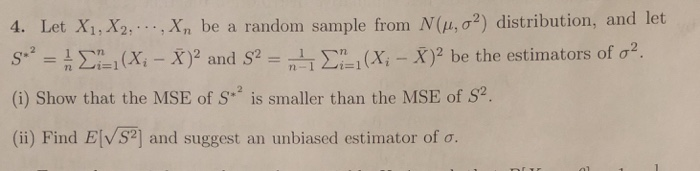4. Let X1,X2, ,Xn be a randonn sample from N(μ, σ2) distribution, and let s* Ση! (Xi-X)2 and S2-n-T Ση#1 (Xi-X)2 be the estimators of σ2 (i) Show that the MSE of s is smaller than the MSE of S2 (ii) Find E [VS2] and suggest an unbiased estimator of σ.

• ### (4 points) Let Xi, , Xn denote a randon sample from a Normal N(μ, 1) distribution, with 11 as the...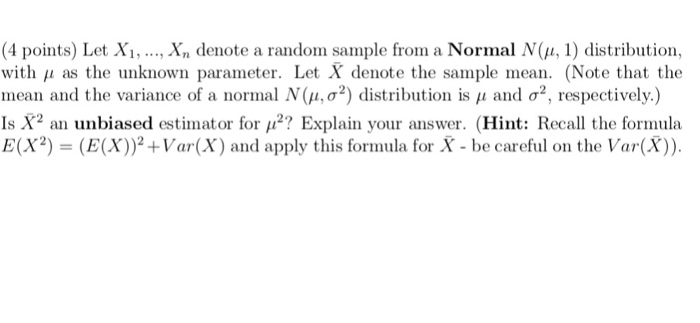please answer with full soultion. with explantion. (4 points) Let Xi, , Xn denote a randon sample from a Normal N(μ, 1) distribution, with 11 as the unknown parameter. Let X denote the sample mean. (Note that the mean and the variance of a normal N(μ, σ2) distribution is μ and σ2, respectively.) Is X2 an unbiased estimator for 112? Explain your answer. (Hint: Recall the fornula E(X2) (E(X)Var(X) and apply this formula for X - be careful on the...

• ### 1. (40) Suppose that X1, X2, Xn forms an independent and identically distributed sample from a normal distribution with mean μ and variance σ2, both unknown: 2nơ2 (a) Derive the sample variance, S2,...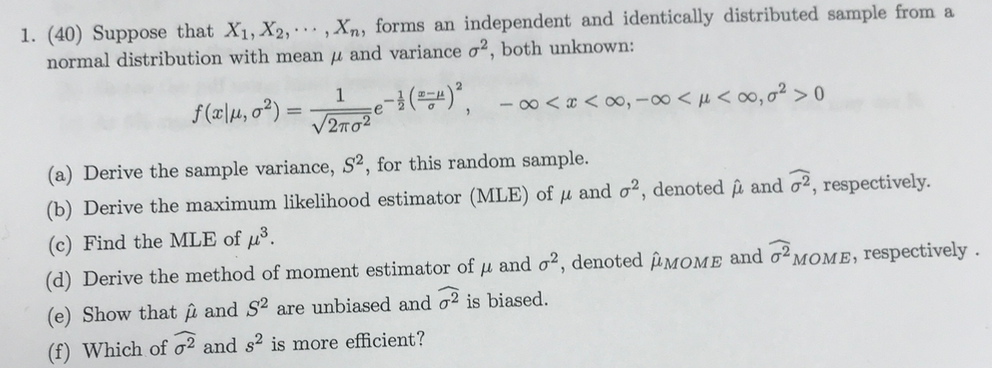1. (40) Suppose that X1, X2, Xn forms an independent and identically distributed sample from a normal distribution with mean μ and variance σ2, both unknown: 2nơ2 (a) Derive the sample variance, S2, for this random sample. (b) Derive the maximum likelihood estimator (MLE) of μ and σ2 denoted μ and σ2, respectively. (c) Find the MLE of μ3 (d) Derive the method of moment estimator of μ and σ2, denoted μΜΟΜΕ and σ2MOME, respectively (e) Show that μ and...

• ### Let X1,.....,Xn be a random sample from N(μ,σ2), and both μ and σ2 are unknown, with...

Let X1,.....,Xn be a random sample from N(μ,σ2), and both μ and σ2 are unknown, with -∞<μ<∞ and σ2 > 0. a. Develop a likelihood ratio test for H0: μ <= μ0 vs. H1: μ > μ0 b. Develop a likelihood ratio test for H0: μ >= μ0 vs. H1: μ < μ0

• ### Let X1,.....,Xn be a random sample from N(μ,σ2), and both μ and σ2 are unknown, with...

Let X1,.....,Xn be a random sample from N(μ,σ2), and both μ and σ2 are unknown, with -∞<μ<∞ and σ2 > 0. a. Develop a likelihood ratio test for H0: μ <= μ0 vs. H1: μ > μ0 b. Develop a likelihood ratio test for H0: μ >= μ0 vs. H1: μ < μ0

• ### 4. Let Xi, , xn be a sample from N(μ, σ2). Use the distributions of sarnple...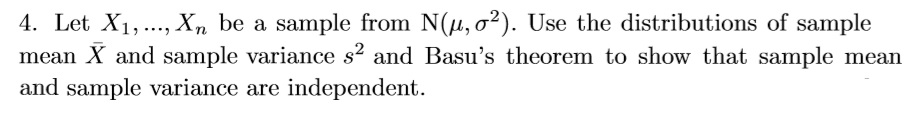4. Let Xi, , xn be a sample from N(μ, σ2). Use the distributions of sarnple mean X and sample variance s2 and Basu's theorem to show that sample mean and sample variance are independent.

• ### 6. Let Xi 1,... ,Xn be a random sample from a normal distribution with mean u and variance ơ2 whi...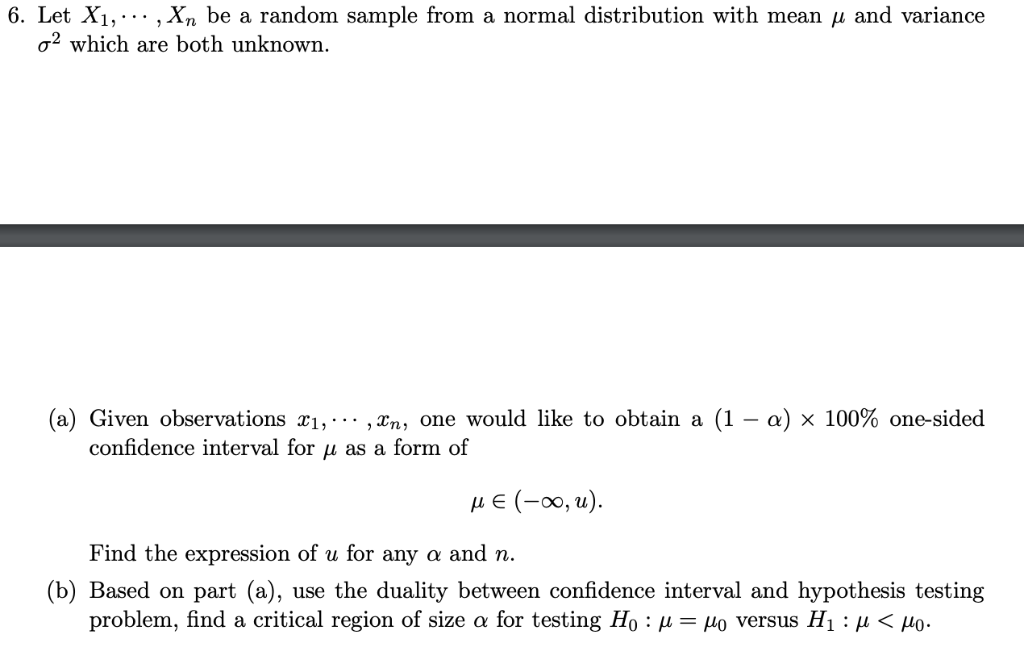6. Let Xi 1,... ,Xn be a random sample from a normal distribution with mean u and variance ơ2 which are both unknown. (a) Given observations xi, ,Xn, one would like to obtain a (1-a) x 100% one-sided confidence interval for u as a form of L E (-00, u) the expression of u for any a and n. (b) Based on part (a), use the duality between confidence interval and hypothesis testing problem, find a critical region of size...